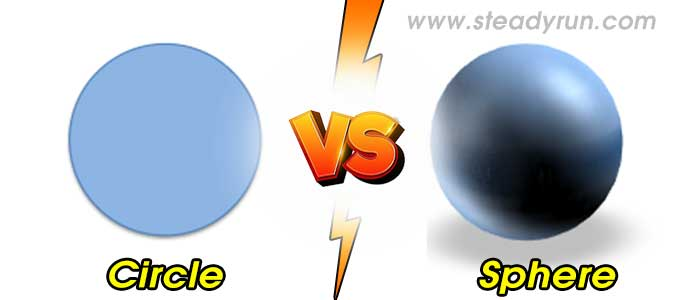# Difference between Circle and SphereDistinguish, differentiate, compare and explain what is the Difference between Circle and Sphere. Comparison and Differences.

Circle

A circle is formed by a point moving around a fixed point (the centre) at a constant distance. A circle is the set of all points in the plane that are a fixed distance (the radius) from a fixed point (the centre).

Any interval joining a point on the circle to the centre is called a radius. By the definition of a circle, any two radii have the same length.

An interval joining two points on the circle is called a chord.

A chord that passes through the centre is called a diameter. Since a diameter consists of two radii joined at their endpoints, every diameter has length equal to twice the radius.

Sphere

A sphere is a three-dimensional form of a circle, where all points on the surface of the sphere are at a constant distance from the centre of the sphere.

The Earth is not exactly a sphere. A grapefruit is a better analogy, as it is flatter at the top and bottom (an oblate sphere). The diameter as measured through the North and South Poles is about 40 km less than the diameter using two points on the Equator. The radius of the Earth is usually given as 6 370 kilometers.

## Difference between Circle and Sphere

1. Circle is a figure, a sphere is an object.

2. A circle is a 2D figure whereas a sphere is a 3D object having volume.

3. One can only calculate the surface area of a circle whereas it is possible to calculate the volume of a sphere.

#### Tags:

Difference between Sphere vs Circle

Circle vs Sphere

Differences between Sphere vs Circle

## Related Categories### Spreading Knowledge Across the World

USA - United States of America  Canada  United Kingdom  Australia  New Zealand  South America  Brazil  Portugal  Netherland  South Africa  Ethiopia  Zambia  Singapore  Malaysia  India  China  UAE - Saudi Arabia  Qatar  Oman  Kuwait  Bahrain  Dubai  Israil  England  Scotland  Norway  Ireland  Denmark  France  Spain  Poland  and  many more....